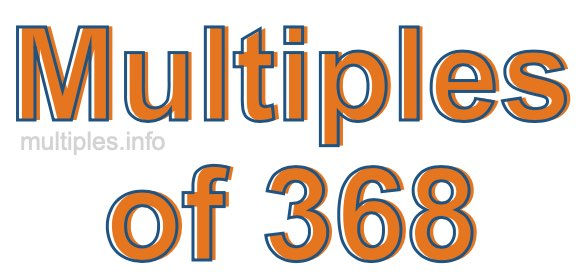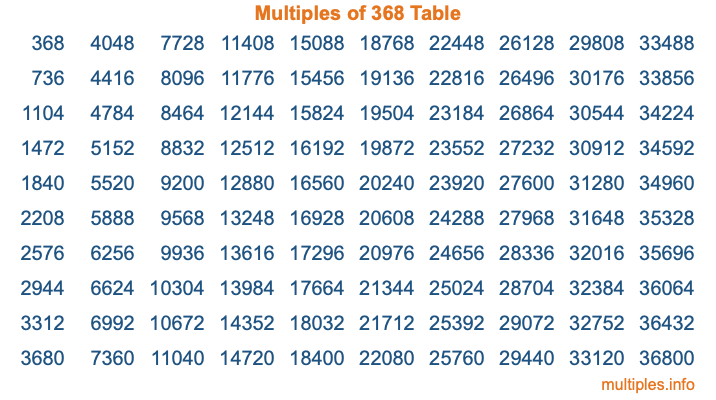Multiples of 368Welcome to the Multiples of 368 page. Here we will first teach you everything you will ever need to know about the multiples of 368, and then give you a study guide summary of everything we taught you to make sure you remember it all. Use this page to look up facts and learn information about the multiples of 368. This page will make you a multiples of three hundred sixty-eight expert!

Definition of Multiples of 368
Multiples of 368 are all the numbers that when divided by 368 equal an integer. Each of the multiples of 368 are called a multiple. A multiple of 368 is created by multiplying 368 by an integer.

Therefore, to create a list of multiples of 368, you start with 1 multiplied by 368, then 2 multiplied by 368, then 3 multiplied by 368, and so on for as long as you want. Thus, the list of the first five multiples of 368 is 368, 736, 1104, 1472, and 1840. To see a larger list of multiples of 368, see the printable image of Multiples of 368 further down on this page. We also have a category where you can choose any nth multiple of 368.

Multiples of 368 Checker
The Multiples of 368 Checker below checks to see if any number of your choice is a multiple of 368. In other words, it checks to see if there is any number (integer) that when multiplied by 368 will equal your number. To do that, we divide your number by 368. If the the quotient is an integer, then your number is a multiple of 368.

Is  a multiple of 368?

Least Common Multiple of 368 and ...
A Least Common Multiple (LCM) is the lowest multiple that two or more numbers have in common. This is also called the smallest common multiple or lowest common multiple and is useful to know when you are adding our subtracting fractions. Enter one or more numbers below (368 is already entered) to find the LCM.

Check out our LCM Calculator if you need more details about the Least Common Multiple or if you need the LCM for different numbers for adding and subtraction fractions.

nth Multiple of 368
As we stated above, 368 is the first multiple of 368, 736 is the second multiple of 368, 1104 is the third multiple of 368, and so on. Enter a number below to find the nth multiple of 368.

th multiple of 368

Multiples of 368 vs Factors of 368
368 is a multiple of 368 and a factor of 368, but that is where the similarities end. All postive multiples of 368 are 368 or greater than 368. All positive factors of 368 are 368 or less than 368.

Below is the beginning list of multiples of 368 and the factors of 368 so you can compare:

Multiples of 368: 368, 736, 1104, 1472, 1840, etc.

Factors of 368: 1, 2, 4, 8, 16, 23, 46, 92, 184, 368

As you can see, the multiples of 368 are all the numbers that you can divide by 368 to get a whole number. The factors of 368, on the other hand, are all the whole numbers that you can multiply by another whole number to get 368.

It's also interesting to note that if a number (x) is a factor of 368, then 368 will also be a multiple of that number (x).

Multiples of 368 vs Divisors of 368
The divisors of 368 are all the integers that 368 can be divided by evenly. Below is a list of the divisors of 368.

Divisors of 368: 1, 2, 4, 8, 16, 23, 46, 92, 184, 368

The interesting thing to note here is that if you take any multiple of 368 and divide it by a divisor of 368, you will see that the quotient is an integer.

Multiples of 368 Table
Below is an image of the first 100 multiples of 368 in a table. The table is in chronological order, column by column. The first column has the first ten multiples of 368, the second column has the next ten multiples of 368, and so on.The Multiples of 368 Table is also referred to as the 368 Times Table or Times Table of 368. You are welcome to print out our table for your studies.

Negative Multiples of 368
Although not often discussed or needed in math, it is worth mentioning that you can make a list of negative multiples of 368 by multiplying 368 by -1, then by -2, then by -3, and so on, to get the following list of negative multiples of 368:

-368, -736, -1104, -1472, -1840, etc.

Multiples of 368 Summary
Below is a summary of important Multiples of 368 facts that we have discussed on this page. To retain the knowledge on this page, we recommend that you read through the summary and explain to yourself or a study partner why they hold true.

There are an infinite number of multiples of 368.

A multiple of 368 divided by 368 will equal a whole number.

368 divided by a factor of 368 equals a divisor of 368.

The nth multiple of 368 is n times 368.

The largest factor of 368 is equal to the first positive multiple of 368.

368 is a multiple of every factor of 368.

368 is a multiple of 368.

A multiple of 368 divided by a divisor of 368 equals an integer.

368 divided by a divisor of 368 equals a factor of 368.

Any integer times 368 will equal a multiple of 368.

Multiples of a Number
Here you can get the multiples of another number, all with the same attention to detail as we did for multiples of 368 on this page.

Multiples of
Multiples of 369
Did you find our page about multiples of three hundred sixty-eight educational? Do you want more knowledge? Check out the multiples of the next number on our list!